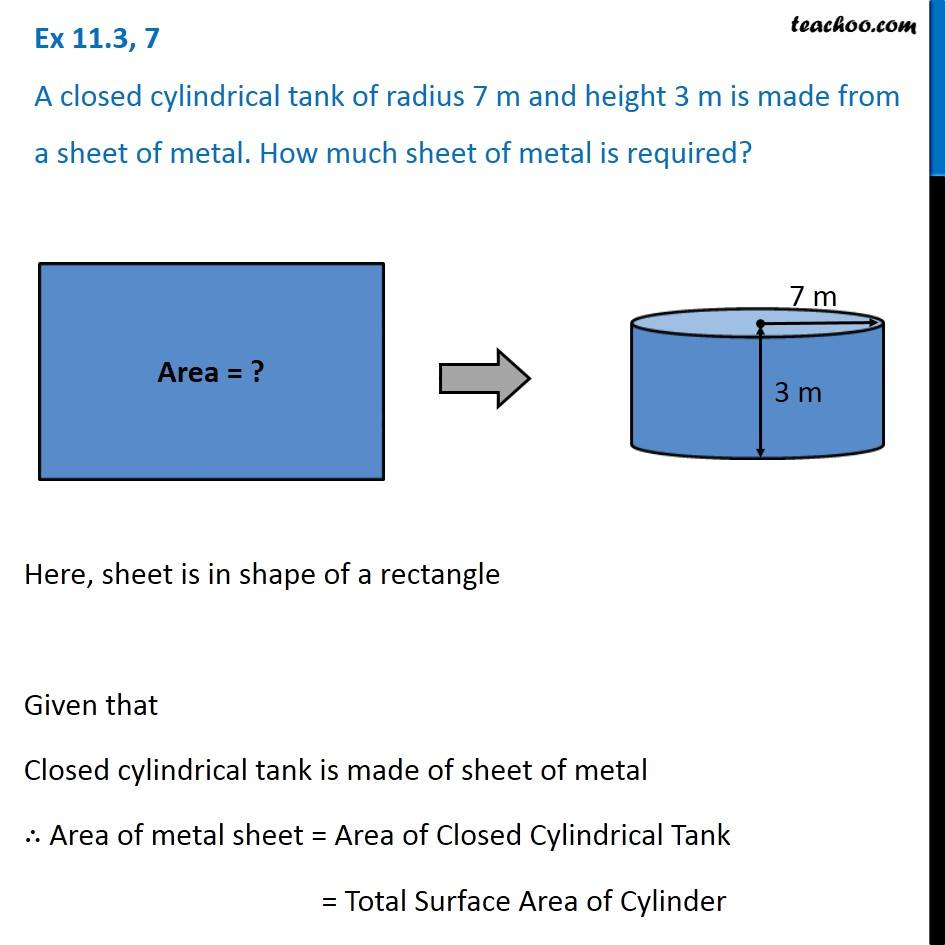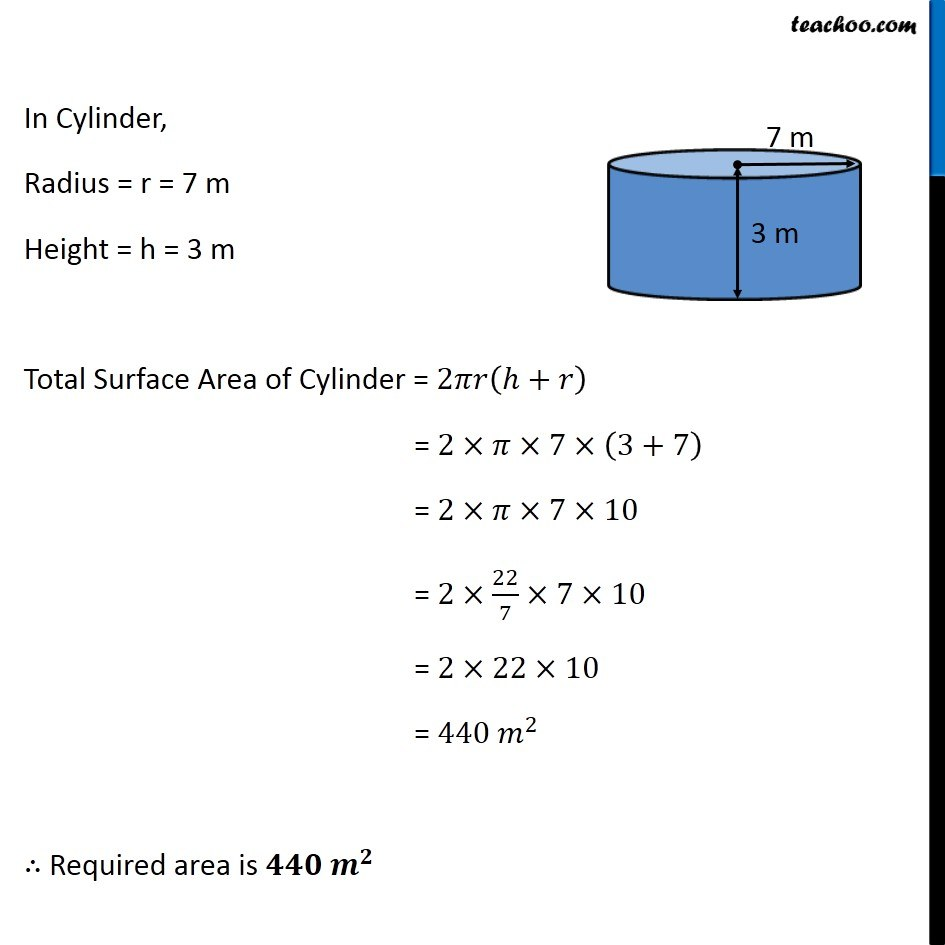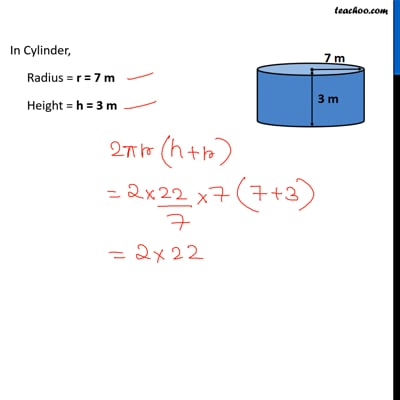Ex 11.3

Chapter 11 Class 8 Mensuration
Serial order wiseThis video is only available for Teachoo black usersThis video is only available for Teachoo black users

Get live Maths 1-on-1 Classs - Class 6 to 12

### Transcript

Ex 11.3, 7 A closed cylindrical tank of radius 7 m and height 3 m is made from a sheet of metal. How much sheet of metal is required? Here, sheet is in shape of a rectangle Given that Closed cylindrical tank is made of sheet of metal ∴ Area of metal sheet = Area of Closed Cylindrical Tank = Total Surface Area of Cylinder In Cylinder, Radius = r = 7 m Height = h = 3 m Total Surface Area of Cylinder = 2𝜋𝑟(ℎ+𝑟) = 2×𝜋×7×(3+7) = 2×𝜋×7×10 = 2×22/7×7×10 = 2×22×10 = 440 𝑚^2 ∴ Required area is 𝟒𝟒𝟎〖 𝒎〗^𝟐